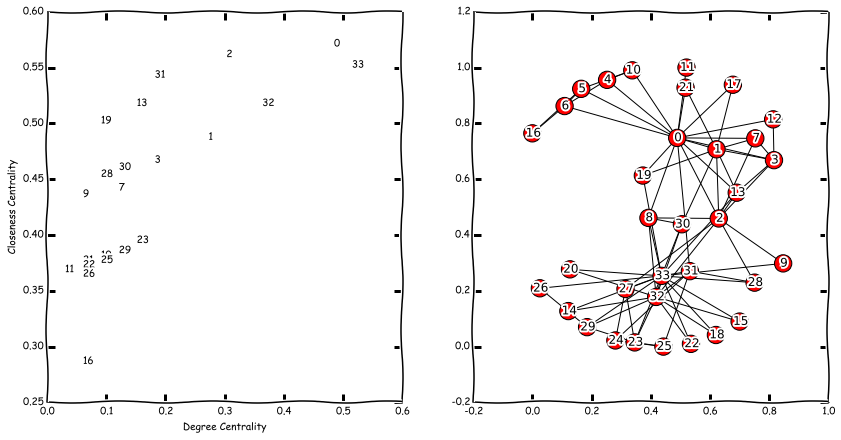# Node and Link analysis: Centrality measures¶

Centrality measures are used to appraise the "importance" of the elements of the network. The problem is that "importance"

• Is not well-defined
• Depends on the domain of the network

During this seminar we will consider two node centrality measures: degree centrality and closeness centrality

## Degree Centrality¶

In fact you have already met the degree centrality in this course.

Given adjacency matrix $A$ of the unweighted and undirected graph $G = (V,E)$ degree centrality of the node $v_i$ is computed as: $$C_D(i) = \sum_j A_{ji}$$ In order to compare nodes across graphs this measure can be normalized by a factor $\frac{1}{N-1}$

## Closeness Centrality¶

The most correspondent to the word "central". Closeness centrality is used to identify nodes that can reach other nodes quickly. $$C_C(i) = \left[ \sum_{j,\ j\neq i} d(v_i, v_j) \right]^{-1}\text{,}$$ where $d(v_i, v_j)$ is a length of the shortest path between $v_i$ and $v_j$. Again, to be normalized it is multiplied by $(N-1)$.

## Why?¶

Centralities allow us to

• Understand the structure of the graph without looking at it
• Compare nodes of a graph (between graphs) and identify the most "important"
• Compare graphs*
In :
import numpy as np
import matplotlib.pyplot as plt
plt.xkcd()
import networkx as nx
%matplotlib inline


## Example: Zachary's Karate Club¶

Let's load Zachary's Karate Club network. This is quite small example so we can both calculate centralities and map them of the picture of the graph

In :
G = nx.karate_club_graph()
pos = nx.spring_layout(G) # Fix node positions on all pictures

In :
# Original network
nx.draw_networkx(G, pos)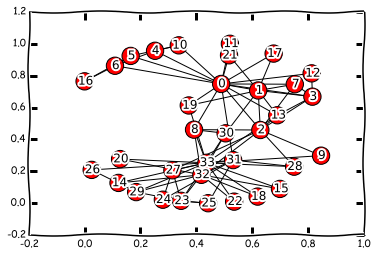In :
# Degree centrality
dc = nx.degree_centrality(G)
plt.figure(1, figsize=(10,7))
coord = nx.spring_layout(G)
nx.draw(G,
pos,
nodelist=dc.keys(),
node_size = [d*7000 for d in dc.values()],
node_color=dc.values(),
font_size=8,
cmap=plt.cm.Reds,
)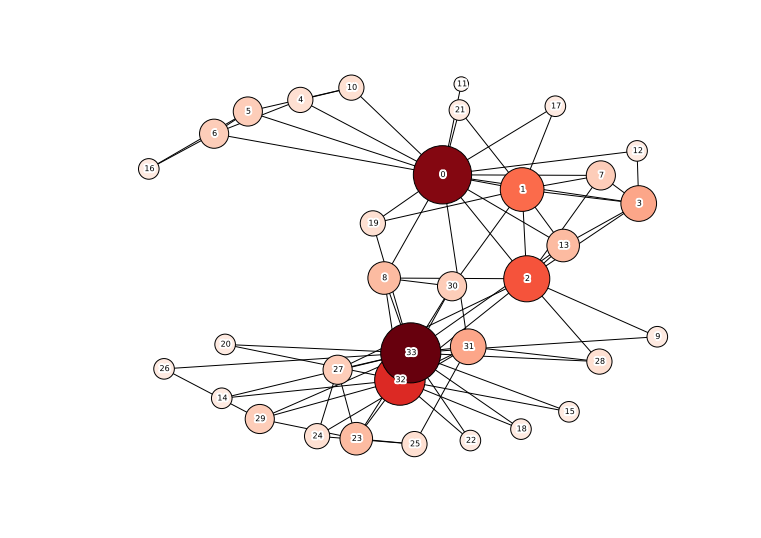In :
# Closeness centrality
cl = nx.closeness_centrality(G)
plt.figure(1, figsize=(10,7))
coord = nx.spring_layout(G)
nx.draw(G,
pos,
nodelist=cl.keys(),
node_size = [d*3000 for d in cl.values()],
node_color=cl.values(),
font_size=8,
cmap=plt.cm.Reds,
)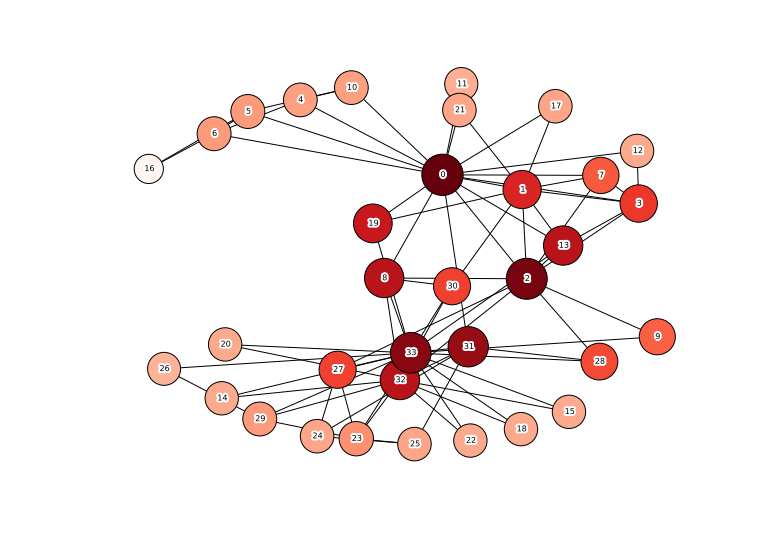In :
# Plot degree-closeness
xdata = dc.values()
ydata = cl.values()
plt.figure(1, figsize=(7,7))
plt.plot(xdata,ydata, '+')
plt.xlabel('Degree Centrality')
plt.ylabel('Closeness Centrality')

Out:
<matplotlib.text.Text at 0xc468710>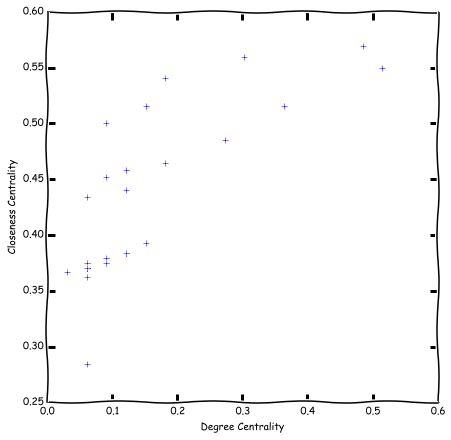In :
# Not Clear. Lets add node ids:
fig = plt.figure(1, figsize=(14,7))